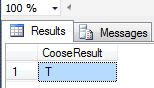# New Choose Function in SQL Server 2012

In this article I have described about new choose function in SQL Server 2012.
•7398

Choose() Function

This function is used to return the value out of a list based on its index number. You can think it as like an array. The Index number here starts from 1.

Syntax

CHOOSE ( index, value1, value2.... [, valueN ] )

CHOOSE() Function excepts two parameters,

Index: Index is an integer expression that represents an index into the list of the items. The list index always starts at 1.

Value: List of values of any data type.

Now some facts related to the Choose Function

1. Item index starts from 1

DECLARE @ShowIndex INT;

SET @ShowIndex =5;

Select Choose(@ShowIndex, 'M','N','H','P','T','L','S','H') As ChooseResult

In the preceding example we take index=5. It will start at 1. Choose() returns T as output since T is present at @Index location 5.

Output2.  When passed a set of types to the function it returns the data type with the highest precedence; see:

DECLARE @ShowIndex INT;

SET @ShowIndex =5;

Select Choose(@ShowIndex ,35,42,12.6,14,15,18.7)  As CooseResult

In this example we use index=5. It will start at 1. Choose() returns 15.0 as output since 15 is present at @ShowIndex location 5 because in the item list, fractional numbers have higher precedence than integers.3. If an index value exceeds the bound of the array it returns NULL

DECLARE @ShowIndex INT;

SET @ShowIndex =9;

Select Choose(@ShowIndex , 'M','N','H','P','T','L','S','H')  As CooseResult

In this example we take index=9. It will start at 1. Choose() returns Null as output because in the item list the index value exceeds the bounds of the array; the last Index=8.

Output